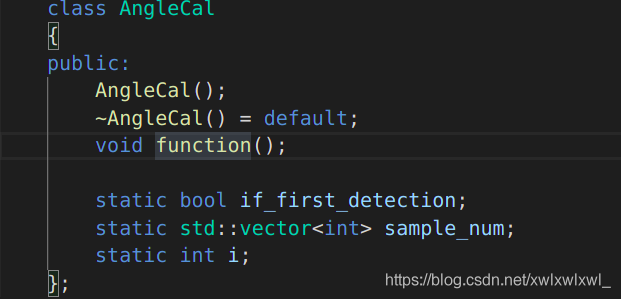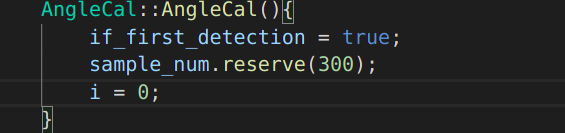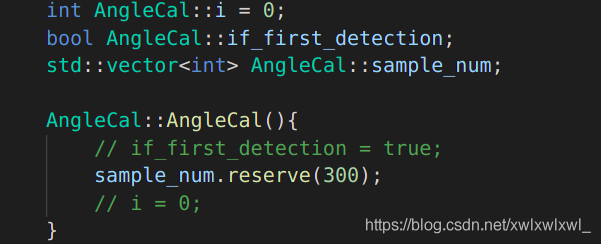# libxx.so: undefined reference, vector.reserve(n) [How to Solve]

background: Trying to avoid reallocation using the STL: std::vector.reserve(n).
declared static std::vector<int> sample_num in class AngleCal, and want to do it in AngleCal::AngleCal() sample_num.reserve(300) (assumming 300 is the size or, alternatively an upper bound on the size)However, when compiling this AngleCal.cpp into libanglecal.so, the compiler gives the following error.
[build] /usr/bin/ld: ../lib/libanglecal.so: undefined reference to `hitcrt::AngleCal::if_first_detection’
[build] /usr/bin/ld: ../lib/libanglecal.so: undefined reference to `hitcrt::AngleCal::sample_num’
[build] /usr/bin/ld: ../lib/libanglecal.so: undefined reference to `hitcrt::AngleCal::i’
Solution: A static member is shared by all objects of the class. All static data is initialized to zero when the first object is created, if no other initialization is present. We can’t put it in the class definition but it can be initialized outside the class as done in the following example by redeclaring the static variable, using the scope resolution operator :: to identify which class it belongs to.

``````#include <iostream>

using namespace std;

class Box {
public:
static int objectCount;

// Constructor definition
Box(double l = 2.0, double b = 2.0, double h = 2.0) {
cout <<"Constructor called." << endl;
length = l;
breadth = b;
height = h;

// Increase every time object is created
objectCount++;
}
double Volume() {
return length * breadth * height;
}

private:
double length;     // Length of a box
double breadth;    // Breadth of a box
double height;     // Height of a box
};

// Initialize static member of class Box
int Box::objectCount = 0;

int main(void) {
Box Box1(3.3, 1.2, 1.5);    // Declare box1
Box Box2(8.5, 6.0, 2.0);    // Declare box2

// Print total number of objects.
cout << "Total objects: " << Box::objectCount << endl;

return 0;
}``````

The running results are as follows

``````Constructor called.
Constructor called.
Total objects: 2``````

Static decorated class members must be initialized outside the class before they can be manipulated.above.